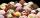# Blocks

There are 9 interactive basic building blocks of an organization. How many two-blocks combinations are there?

Result

n =  36

#### Solution:Leave us a comment of example and its solution (i.e. if it is still somewhat unclear...):

Showing 0 comments:Be the first to comment!#### To solve this example are needed these knowledge from mathematics:

Would you like to compute count of combinations?

## Next similar examples:

1. Three workshopsThere are 2743 people working in three workshops. In the second workshop works 140 people more than in the first and in third works 4.2 times more than the second one. How many people work in each workshop?
2. TeamsHow many ways can divide 16 players into two teams of 8 member?
3. Fish tankA fish tank at a pet store has 8 zebra fish. In how many different ways can George choose 2 zebra fish to buy?
4. ChordsHow many 4-tones chords (chord = at the same time sounding different tones) is possible to play within 7 tones?
5. ExaminationThe class is 21 students. How many ways can choose two to examination?
6. TrinityHow many different triads can be selected from the group 43 students?
7. The confectioneryThe confectionery sold 5 kinds of ice cream. In how many ways can I buy 3 kinds if order of ice creams does not matter?
8. ConfectioneryThe village markets have 5 kinds of sweets, one weighs 31 grams. How many different ways a customer can buy 1.519 kg sweets.
9. Weekly serviceIn the class are 20 pupils. How many opportunities have the teacher if he wants choose two pupils randomly who will weeklies?
10. Volleyball8 girls wants to play volleyball against boys. On the field at one time can be six players per team. How many initial teams of this girls may trainer to choose?
11. Theorem proveWe want to prove the sentense: If the natural number n is divisible by six, then n is divisible by three. From what assumption we started?
12. Calculation of CNCalculate: ?
13. Count of trianglesGiven a square ABCD and on each side 8 internal points. Determine the number of triangles with vertices at these points.
14. BallsThe urn is 8 white and 6 black balls. We pull 4 randomly balls. What is the probability that among them will be two white?
15. LineIt is true that the lines that do not intersect are parallel?
16. Reference angleFind the reference angle of each angle:
17. SequenceWrite the first 7 members of an arithmetic sequence: a1=-3, d=6.